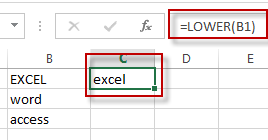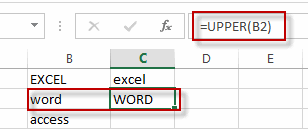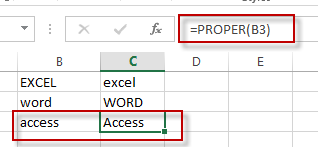# Change Uppercase and Lowercase Text

This post will guide you how to change the case of text to uppercase or lowercase in Excel. How do I change all uppercase text to lower case with an Excel formula.

For example, if you want to change all text string in uppercase into lowercase, you can create a formula based on the Lower function. And if you want to convert all letters in one cell into uppercase, you can use the Upper function as a formula in excel.

If you want to show in Cell C1 a lowercase version of the text in Cell B1, you can type the following formula into the formula box in Cell C1.

=LOWER(B1)If you want to show in Cell C2 an uppercase version of the text in B2, you could type the below formula into the formula box in C2.

=UPPER(B2)If you want to show in Cell C3 a proper version of the text in B3, you could type the below formula into the formula box in C3:

=PROPER(B3)### Related Functions

• Excel UPPER function
The Excel UPPER function converts all characters in text string to uppercase.The UPPER function is a build-in function in Microsoft Excel and it is categorized as a Text Function.The syntax of the UPPER function is as below:= UPPER (text)…
• Excel LOWER function
The Excel LOWER function converts all characters in a text string to lowercase.The syntax of the LOWER function is as below:= LOWER  (text)…
• Excel Proper Function
The Excel PROPER function capitalizes the first character in each word of a text string and set other characters to lowercase. The syntax of the PROPER function is as below:= PROPER  (text)…